### Lighthouse Step 1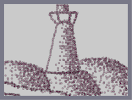Hover over the thumbnail for a full-size version.

Author cucumber_boy art author:cucumber_boy lighthouse n-art nart nonplayable rated 2007-08-28 3 by 5 people. \$Lighthouse Step 1#cucumber_boy#none#00000000000000000000000000000000000000000000000000000000000000000000000000000000000000000000000000000000000000000000000000000000000000000000000000000000000000000000000000000000000000000000000000000000000000000000000000000000000000000000000000000000000000000000000000000000000000000000000000000000000000000000000000000000000000000000000000000000000000000000000000000000000000000000000000000000000000000000000000000000000000000000000000000000000000000000000000000000000000000000000000000000000000000000000000000000000000000000000000000000000000000000000000000000000000000000000000000000000000000000000000000000000000000000000000000000000000000000000000000000000000000000000000000000000000000000000000000000000000000|3^342,151!3^335,179!3^327,211!3^317,247!3^311,286!3^303,330!3^291,368!3^278,423!3^434,150!3^449,248!3^441,201!3^454,294!3^464,333!3^469,375!3^356,139!3^373,134!3^390,133!3^406,138!3^424,144!3^436,176!3^443,225!3^452,271!3^457,313!3^465,354!3^473,398!3^478,423!3^336,166!3^332,195!3^321,229!3^315,269!3^304,310!3^296,351!3^286,390!3^283,408!3^437,159!3^347,146!3^377,133!3^394,133!3^404,134!3^416,143!3^439,150!3^437,171!3^437,188!3^434,158!3^434,177!3^434,189!3^440,202!3^440,230!3^444,244!3^448,254!3^450,267!3^435,196!3^438,216!3^438,216!3^445,258!3^446,266!3^446,268!3^453,283!3^453,296!3^455,302!3^455,323!3^456,358!3^453,342!3^462,360!3^465,362!3^463,343!3^466,385!3^466,403!3^475,422!3^470,414!3^307,100!3^315,115!3^329,132!3^298,86!3^300,78!3^318,78!3^342,78!3^360,78!3^378,78!3^396,78!3^414,78!3^432,78!3^456,78!3^443,138!3^451,119!3^459,99!3^465,77!3^298,435!3^320,438!3^343,442!3^364,442!3^391,440!3^420,438!3^442,436!3^466,430!3^490,370!3^534,355!3^572,354!3^607,354!3^637,354!3^668,363!3^698,375!3^717,386!3^745,396!3^36,375!3^53,369!3^74,371!3^100,373!3^127,383!3^149,384!3^184,387!3^220,396!3^245,409!3^281,412!3^282,399!3^288,379!3^294,359!3^299,340!3^303,318!3^308,295!3^304,303!3^311,277!3^316,259!3^317,237!3^324,217!3^326,204!3^334,189!3^338,161!3^389,174!3^402,226!3^422,305!3^430,326!3^444,373!3^411,258!3^401,171!3^410,231!3^435,289!3^442,389!3^418,156!3^435,202!3^421,221!3^428,236!3^440,286!3^452,325!3^452,379!3^421,177!3^420,218!3^420,232!3^419,259!3^425,330!3^438,356!3^384,188!3^384,232!3^384,290!3^395,370!3^420,388!3^405,223!3^396,256!3^394,303!3^418,354!3^434,402!3^450,418!3^420,192!3^420,240!3^420,300!3^432,336!3^432,360!3^456,408!3^396,192!3^396,216!3^408,276!3^408,300!3^432,348!3^432,264!3^432,276!3^444,348!3^444,312!3^408,168!3^408,204!3^408,216!3^420,276!3^420,348!3^432,372!3^432,204!3^432,216!3^444,228!3^444,252!3^444,288!3^444,288!3^456,312!3^456,324!3^456,324!3^456,348!3^456,384!3^468,396!3^468,396!3^420,156!3^420,192!3^420,216!3^420,264!3^420,312!3^444,336!3^444,372!3^444,396!3^480,408!3^420,156!3^396,180!3^396,252!3^396,300!3^396,300!3^420,408!3^372,168!3^372,216!3^372,276!3^372,312!3^384,360!3^408,432!3^384,408!3^420,180!3^420,216!3^420,288!3^432,324!3^432,348!3^444,372!3^444,396!3^444,384!3^444,360!3^420,156!3^420,192!3^432,300!3^444,312!3^444,324!3^396,240!3^432,252!3^432,252!3^408,252!3^408,240!3^408,252!3^420,252!3^432,240!3^420,204!3^408,204!3^408,168!3^408,204!3^408,264!3^420,276!3^444,312!3^444,324!3^408,264!3^408,300!3^420,336!3^432,360!3^432,372!3^432,384!3^444,408!3^444,408!3^396,156!3^384,228!3^384,276!3^384,360!3^396,372!3^360,360!3^384,192!3^384,216!3^384,216!3^384,336!3^396,384!3^408,384!3^396,336!3^408,372!3^408,408!3^348,420!3^408,180!3^408,204!3^420,168!3^420,240!3^408,360!3^408,312!3^408,336!3^408,360!3^420,384!3^420,396!3^420,408!3^444,408!3^420,420!3^432,420!3^396,324!3^408,324!3^432,312!3^396,276!3^396,288!3^408,288!3^396,300!3^396,288!3^396,288!3^396,288!3^408,264!3^408,264!3^384,282!3^420,282!3^414,282!3^408,192!3^444,300!3^420,300!3^420,288!3^396,180!3^396,276!3^396,336!3^372,396!3^372,396!3^360,336!3^348,408!3^360,252!3^360,276!3^348,408!3^396,192!3^396,204!3^403,187!3^403,199!3^404,199!3^425,204!3^422,241!3^420,241!3^422,309!3^422,329!3^422,373!3^422,378!3^433,387!3^453,402!3^376,255!3^376,274!3^436,269!3^436,326!3^436,353!3^452,370!3^418,412!3^448,412!3^448,422!3^407,349!3^406,360!3^416,405!3^420,414!3^396,402!3^398,416!3^482,367!3^501,358!3^515,358!3^517,357!3^532,355!3^543,355!3^565,354!3^575,353!3^592,353!3^620,346!3^631,361!3^659,362!3^690,363!3^705,380!3^735,396!3^763,412!3^492,362!3^516,352!3^558,353!3^594,352!3^612,350!3^652,352!3^680,363!3^715,382!3^756,405!3^764,411!3^507,362!3^530,355!3^571,356!3^598,354!3^624,353!3^634,353!3^634,353!3^634,353!3^669,357!3^709,374!3^735,389!3^741,398!3^764,412!3^29,374!3^46,374!3^65,373!3^93,374!3^112,377!3^135,383!3^163,392!3^163,392!3^215,400!3^252,421!3^273,426!3^185,390!3^212,407!3^218,412!3^279,427!3^281,433!3^292,433!3^300,435!3^307,436!3^312,436!3^318,436!3^328,439!3^340,441!3^343,439!3^354,440!3^370,441!3^371,440!3^388,440!3^402,439!3^413,439!3^417,437!3^440,438!3^441,438!3^455,436!3^470,434!3^470,433!3^434,163!3^436,188!3^436,188!3^436,188!3^447,230!3^440,246!3^440,248!3^449,254!3^448,282!3^448,311!3^448,330!3^451,343!3^468,362!3^468,363!3^469,404!3^471,414!3^471,414!3^466,404!3^466,404!3^453,291!3^454,292!3^455,358!3^456,380!3^466,402!3^174,396!3^191,397!3^212,405!3^219,410!3^253,426!3^43,373!3^74,374!3^77,374!3^77,374!3^121,383!3^142,389!3^189,391!3^202,391!3^215,409!3^235,413!3^235,413!3^254,425!3^259,433!3^298,88!3^299,91!3^299,91!3^308,112!3^330,125!3^330,136!3^462,86!3^459,86!3^456,110!3^445,136!3^447,129!3^431,155!3^455,102!3^449,120!3^444,133!3^306,78!3^312,78!3^318,78!3^324,78!3^342,78!3^312,72!3^330,72!3^330,72!3^342,72!3^354,72!3^360,72!3^372,72!3^378,72!3^390,72!3^402,72!3^402,72!3^420,72!3^420,72!3^420,72!3^444,72!3^450,72!3^342,78!3^348,78!3^360,78!3^378,78!3^378,78!3^396,78!3^408,78!3^414,78!3^426,78!3^426,78!3^438,78!3^444,78!3^444,78!3^336,78!3^384,78!3^369,77!3^342,84!3^348,99!3^348,104!3^348,113!3^351,115!3^364,131!3^355,124!3^346,90!3^418,89!3^417,105!3^417,113!3^417,129!3^417,128!3^417,100!3^417,100!3^416,121!3^417,88!3^342,42!3^342,54!3^342,66!3^342,30!3^408,60!3^408,48!3^408,36!3^408,36!3^360,30!3^372,30!3^390,30!3^390,30!3^390,30!3^342,30!3^348,30!3^372,30!3^384,30!3^402,30!3^402,30!3^354,378!3^339,138!3^320,122!3^384,441!3^386,441!3^397,441!3^419,446!3^444,443!3^465,437!3^466,432!3^391,73!3^424,76!3^426,76!3^443,75!3^455,75!3^458,86!3^456,93!3^448,118!3^447,118!3^424,90!3^424,95!3^423,95!3^422,135!3^422,115!3^351,88!3^351,104!3^352,107!3^352,116!3^361,125!3^361,134!3^374,134!3^375,129!3^387,122!3^402,123!3^402,135!3^403,141!3^421,143!3^423,143!3^389,128!3^410,129!3^410,143!3^273,460!3^289,496!3^292,514!3^300,544!3^489,424!3^520,438!3^534,448!3^554,466!3^570,488!3^570,509!3^586,530!3^598,549!3^39,497!3^66,499!3^93,510!3^108,516!3^139,517!3^179,532!3^210,533!3^239,535!3^249,537!3^270,548!3^26,558!3^54,563!3^81,573!3^99,574!3^117,574!3^434,440!3^263,439!3^271,458!3^271,458!3^289,489!3^289,493!3^289,511!3^290,524!3^298,539!3^261,446!3^271,453!3^277,470!3^279,473!3^285,485!3^286,487!3^287,510!3^306,551!3^501,427!3^503,429!3^522,440!3^522,446!3^531,447!3^541,470!3^552,483!3^569,494!3^572,508!3^583,513!3^591,529!3^591,534!3^592,539!3^595,552!3^593,566!3^598,566!3^498,427!3^512,427!3^517,441!3^534,446!3^540,462!3^545,463!3^546,463!3^566,494!3^566,511!3^586,525!3^590,538!3^591,542!3^37,491!3^56,498!3^73,509!3^73,513!3^105,519!3^106,519!3^129,519!3^154,519!3^156,520!3^171,520!3^188,530!3^202,537!3^222,537!3^234,536!3^253,538!3^280,538!3^285,545!3^298,550!3^319,551!3^320,551!3^320,551!3^320,551!3^333,551!3^350,550!3^351,550!3^353,555!3^367,565!3^379,565!3^394,565!3^397,565!3^421,566!3^422,566!3^422,566!3^35,565!3^45,565!3^58,566!3^25,561!3^32,563!3^33,563!3^56,565!3^68,566!3^81,567!3^83,566!3^83,565!3^31,496!3^39,497!3^71,499!3^110,518!3^140,532!3^166,532!3^196,531!3^256,553!3^302,559!3^384,557!3^418,570!3^444,573!3^35,370!3^45,369!3^69,366!3^120,369!3^206,392!3^232,406!3^255,424!3^256,436!3^135,375!3^157,380!3^184,384!3^207,396!3^227,411!3^239,419!3^274,451!3^292,483!3^293,490!3^295,523!3^552,349!3^594,363!3^596,363!3^652,371!3^704,369!3^719,386!3^740,414!3^753,414!3^637,347!3^688,363!3^711,372!3^727,390!10^289,437!10^309,438!10^330,438!10^353,438!10^362,437!10^362,437!10^449,439!3^330,433!3^353,436!3^383,437!3^383,435!3^383,434!3^363,446!3^364,446!3^364,446!3^444,439!3^444,438!3^615,384!3^656,423!3^677,493!3^683,525!3^600,354!3^630,355!3^655,359!3^663,371!3^684,382!3^685,390!3^701,399!3^737,417!3^755,417!3^568,405!3^623,403!3^667,418!3^702,441!3^738,469!3^518,410!3^558,439!3^562,461!3^600,534!3^628,555!3^527,389!3^584,399!3^621,444!3^653,513!3^670,549!3^690,565!3^606,466!3^642,509!3^647,382!3^710,380!3^726,398!3^745,416!3^724,432!3^570,372!3^596,372!3^635,376!3^635,377!3^635,382!3^671,400!3^709,417!3^722,426!3^732,438!3^761,450!3^595,389!3^642,395!3^676,414!3^695,416!3^716,434!3^738,451!3^738,463!3^752,465!3^755,487!3^564,384!3^600,384!3^648,396!3^720,420!3^756,444!3^756,444!3^768,492!3^600,408!3^660,432!3^660,432!3^684,456!3^696,468!3^708,480!3^732,504!3^744,516!3^744,540!3^744,552!3^744,552!3^708,432!3^732,468!3^744,480!3^24,492!3^48,492!3^48,504!3^60,504!3^84,504!3^108,528!3^156,528!3^156,528!3^204,528!3^30,498!3^48,498!3^48,498!3^72,498!3^84,516!3^96,516!3^114,516!3^132,516!3^144,516!3^144,522!3^162,534!3^186,534!3^198,534!3^222,534!3^240,534!3^240,534!3^252,534!3^270,534!3^312,552!3^348,552!3^348,552!3^348,552!3^348,552!3^372,558!3^402,552!3^42,564!3^66,564!3^72,564!3^96,570!3^108,576!3^114,576!3^101,375!3^123,375!3^145,377!3^159,386!3^180,399!3^230,433!3^234,452!3^248,488!3^268,430!3^514,384!3^544,415!3^605,481!3^637,523!3^572,413!3^608,418!3^637,436!3^651,463!3^670,490!3^677,515!3^680,535!3^684,549!3^571,362!3^623,362!3^636,364!3^674,386!3^703,401!3^718,403!3^723,421!3^751,444!3^749,461!3^682,443!3^695,452!3^696,445!3^721,488!3^537,369!3^568,379!3^608,378!3^669,398!3^694,426!3^728,453!3^728,485!3^755,527!3^677,482!3^689,477!3^726,545!3^737,562!3^587,352!3^642,382!3^675,400!3^700,401!3^706,401!3^734,429!3^747,448!3^756,464!3^764,504!3^639,377!3^658,374!3^683,392!3^720,409!3^720,415!3^740,433!3^754,446!3^672,374!3^742,418!3^601,357!3^629,366!3^641,377!3^664,387!3^686,422!3^700,445!3^730,460!3^747,485!3^750,496!3^773,550!3^645,389!3^684,392!3^685,402!3^685,416!3^551,387!3^553,393!3^566,363!3^566,362!3^588,360!3^612,372!3^636,360!3^660,384!3^684,384!3^684,384!3^684,384!3^684,372!3^696,372!3^720,396!3^720,396!3^696,384!3^708,396!3^732,408!3^744,408!3^720,432!3^744,420!3^768,420!3^768,432!3^744,420!3^744,420!3^744,420!3^612,372!3^612,372!3^624,372!3^624,396!3^624,396!3^624,408!3^624,420!3^624,432!3^660,408!3^660,396!3^540,360!3^552,384!3^588,384!3^612,384!3^624,408!3^624,408!3^648,420!3^648,432!3^660,456!3^672,408!3^672,468!3^672,480!3^684,408!3^684,468!3^684,480!3^708,432!3^696,492!3^720,408!3^720,492!3^720,504!3^744,432!3^744,516!3^744,552!3^528,372!3^540,372!3^540,372!3^348,36!3^348,48!3^348,48!3^348,48!3^396,36!3^396,48!3^396,48!3^408,60!3^408,60!3^384,60!3^384,60!3^396,60!3^384,48!3^384,36!3^360,60!3^372,48!3^389,47!3^398,53!3^398,53!3^398,53!3^385,41!3^393,43!3^398,45!3^404,45!3^403,65!3^396,57!3^397,60!3^408,61!3^408,59!3^369,34!3^385,34!3^388,34!3^406,34!3^406,35!3^406,52!3^406,68!3^406,71!3^383,63!3^595,386!3^660,398!3^679,416!3^699,439!3^713,459!3^726,476!3^739,492!3^749,514!3^475,387!3^491,382!3^494,382!3^532,383!3^570,426!3^606,457!3^624,494!3^649,544!3^574,463!3^599,492!3^612,555!3^574,431!3^597,425!3^637,459!3^675,484!3^702,508!3^545,368!3^605,388!3^639,396!3^639,401!3^670,422!3^698,423!3^729,448!3^755,468!3^550,370!3^585,372!3^585,374!3^666,373!3^725,412!3^532,396!3^589,397!3^603,408!3^622,411!3^645,415!3^665,433!3^679,446!3^720,468!3^734,487!3^747,495!3^753,530!3^612,390!3^662,392!3^704,420!3^722,433!3^597,353!3^659,363!3^694,400!3^714,405!3^731,412!3^753,438!3^759,454!3^480,383!3^498,381!3^529,381!3^537,412!3^617,452!3^641,471!3^653,496!3^486,437!3^493,437!3^507,453!3^512,473!3^531,493!3^543,524!3^493,428!3^519,433!3^520,434!3^521,434!3^522,434!3^539,447!3^539,470!3^558,487!3^562,493!3^574,499!3^588,533!3^588,546!3^588,563!3^591,565!3^541,452!3^541,464!3^564,485!3^585,518!3^595,534!3^601,559!3^497,430!3^515,437!3^449,465!3^463,494!3^485,538!3^483,557!3^476,459!3^503,494!3^515,544!3^520,549!3^427,462!3^449,482!3^450,508!3^471,555!3^472,564!3^301,478!3^308,496!3^324,525!3^337,497!3^336,471!3^360,501!3^376,530!3^388,453!3^409,506!3^455,571!3^359,481!3^392,486!3^394,485!3^430,517!3^512,542!3^494,444!3^539,475!3^547,499!3^549,515!3^550,473!3^557,482!3^566,501!3^588,533!3^589,543!3^598,570!3^495,513!3^525,525!3^556,548!3^574,575!3^573,569!3^463,450!3^471,448!3^523,454!3^522,454!3^523,485!3^544,523!3^556,535!3^449,464!3^468,487!3^496,517!3^522,476!3^519,474!3^540,455!3^636,348!3^688,368!3^703,379!3^713,385!3^718,385!3^720,385!3^720,385!3^720,385!3^756,409!3^492,447!3^509,474!3^526,486!3^544,534!3^544,551!3^475,477!3^507,485!3^532,483!3^490,508!3^539,506!3^561,525!3^562,525!3^408,572!3^541,568!3^536,568!3^463,446!3^453,449!3^502,468!3^500,469!3^499,467!3^553,467!3^470,460!3^451,458!3^517,460!3^480,455!3^456,467!3^553,450!3^622,499!3^559,528!3^479,485!3^530,491!3^529,488!3^577,485!3^443,483!3^484,503!3^486,514!3^595,511!3^592,538!3^463,536!3^545,536!3^509,474!3^525,475!3^546,489!3^548,537!3^560,537!3^503,460!3^504,462!3^527,486!3^486,471!3^507,501!3^510,505!3^510,518!3^519,541!3^525,496!3^523,496!3^498,438!3^536,471!3^537,527!3^556,550!3^568,551!3^568,550!3^565,532!3^534,566!3^558,560!3^510,564!3^522,564!3^503,552!3^487,490!3^548,500!3^560,515!3^486,444!3^493,485!3^532,528!3^487,453!3^487,485!3^519,514!3^546,542!3^555,561!3^481,450!3^500,455!3^513,456!3^529,469!3^530,477!3^553,523!3^582,549!3^582,557!3^465,477!3^493,435!3^493,440!3^506,472!3^516,473!3^537,506!3^538,514!3^499,433!3^531,466!3^540,487!3^565,518!3^477,495!3^510,510!3^511,523!3^521,529!3^524,530!3^524,530!3^527,551!3^537,552!3^567,509!3^576,512!3^578,510!3^480,468!3^504,468!3^504,468!3^504,468!3^540,492!3^540,492!3^552,504!3^564,516!3^576,516!3^576,540!3^576,540!3^528,540!3^492,468!3^516,504!3^528,528!3^456,468!3^492,468!3^528,516!3^540,516!3^540,540!3^504,540!3^528,564!3^564,552!3^564,552!3^504,504!3^504,504!3^372,468!3^396,468!3^396,468!3^432,468!3^456,468!3^408,468!3^444,468!3^396,492!3^444,492!3^396,492!3^444,492!3^420,516!3^468,516!3^480,516!3^468,528!3^492,540!3^444,540!3^480,540!3^432,492!3^432,492!3^468,516!3^480,528!3^504,528!3^576,528!3^432,444!3^444,444!3^444,492!3^444,492!3^444,492!3^492,504!3^504,492!3^528,516!3^540,552!3^492,564!3^492,564!3^504,576!3^504,576!3^516,576!3^540,576!3^744,504!3^744,504!3^756,528!3^756,540!3^746,452!3^745,474!3^745,500!3^760,537!3^759,538!3^757,538!3^678,466!3^700,492!3^721,541!3^738,563!3^740,566!3^755,561!3^756,525!3^755,501!3^752,433!3^765,443!3^764,446!3^507,492!3^527,517!3^426,460!3^417,494!3^419,494!3^398,453!3^442,453!3^464,469!3^477,516!3^507,563!3^524,562!3^587,560!3^587,562!3^594,563!3^444,512!3^445,512!3^461,553!3^470,555!3^477,557!3^411,472!3^424,472!3^415,452!3^466,456!3^488,504!3^521,538!3^544,570!3^546,568!3^573,567!3^574,568!3^441,494!3^464,527!3^449,524!3^439,528!3^428,457!3^478,538!3^491,573!3^509,568!3^569,564!3^570,566!3^396,467!3^431,517!3^431,546!3^332,465!3^369,500!3^397,561!3^420,573!3^411,521!3^455,555!3^385,463!3^385,469!3^404,532!3^430,548!3^424,473!3^464,510!3^484,552!3^387,449!3^434,499!3^452,540!3^473,567!3^425,474!3^485,530!3^494,541!3^389,519!3^449,515!3^493,561!3^409,480!3^511,437!3^510,464!3^556,515!3^404,481!3^451,534!3^467,562!3^598,381!3^647,408!3^685,430!3^708,465!3^721,469!3^748,507!3^765,558!3^755,571!3^748,427!3^748,459!3^513,366!3^513,364!3^526,361!3^526,361!3^683,377!3^700,406!3^722,493!3^734,523!3^734,521!3^688,513!3^719,517!3^730,521!3^658,528!3^679,549!3^716,555!3^760,469!3^762,491!3^775,533!3^442,492!3^500,517!3^508,540!3^144,411!3^190,424!3^201,440!3^239,461!3^274,499!3^271,522!3^194,513!3^236,516!3^161,477!3^201,479!3^103,462!3^189,473!3^35,409!3^71,471!3^74,408!3^94,448!3^101,452!3^147,443!3^168,469!3^184,491!3^177,426!3^118,451!3^163,451!3^198,513!3^33,453!3^113,417!3^73,451!3^113,475!3^129,489!3^131,501!3^60,392!3^92,402!3^123,421!3^204,447!3^246,446!3^262,474!3^275,499!3^190,409!3^230,443!3^253,476!3^267,496!3^199,422!3^240,473!3^240,487!3^239,513!3^155,469!3^209,479!3^212,479!3^226,512!3^180,454!3^204,462!3^221,472!3^145,408!3^184,419!3^187,436!3^208,464!3^137,402!3^199,405!3^233,430!3^252,451!3^282,488!3^153,407!3^195,409!3^222,438!3^238,465!3^261,488!3^202,504!3^236,512!3^205,483!3^227,491!3^227,492!3^242,504!3^150,431!3^152,427!3^169,425!3^199,427!3^225,431!3^230,443!3^253,472!3^266,492!3^224,500!3^241,511!3^277,516!3^165,504!3^165,502!3^186,426!3^170,443!3^206,453!3^217,443!3^219,449!3^167,416!3^119,412!3^189,419!3^226,442!3^256,497!3^260,518!3^191,495!3^210,509!3^237,519!3^247,522!3^162,489!3^177,500!3^177,509!3^109,407!3^128,435!3^143,454!3^142,455!3^141,455!3^153,486!3^150,502!3^126,454!3^131,463!3^131,476!3^131,477!3^123,385!3^135,385!3^170,410!3^196,419!3^215,417!3^224,450!3^234,470!3^235,480!3^247,495!3^265,518!3^212,420!3^212,439!3^247,434!3^261,460!3^262,469!3^262,493!3^221,511!3^230,512!3^147,435!3^146,435!3^142,482!3^143,486!3^141,512!3^179,452!3^177,483!3^177,488!3^217,503!3^236,471!3^215,420!3^226,431!3^228,437!3^244,466!3^262,501!3^270,519!3^270,519!3^166,431!3^194,463!3^194,478!3^232,497!3^246,425!3^246,451!3^247,501!3^29,386!3^75,387!3^79,386!3^86,386!3^110,386!3^121,403!3^129,405!3^155,406!3^164,406!3^171,417!3^191,421!3^202,438!3^204,449!3^227,473!3^227,476!3^228,493!3^245,521!3^218,518!3^235,518!3^137,422!3^143,435!3^187,467!3^191,467!3^112,384!3^117,384!3^161,404!3^183,403!3^230,436!3^236,440!3^255,414!3^254,415!3^254,430!3^269,439!3^270,439!3^272,439!3^272,478!3^272,482!3^275,510!3^287,514!3^285,518!3^241,426!3^284,469!3^283,482!3^291,503!3^291,517!3^291,523!3^187,468!3^144,396!3^168,420!3^192,420!3^168,420!3^192,420!3^216,432!3^144,444!3^180,444!3^192,456!3^168,456!3^216,444!3^156,492!3^168,492!3^192,516!3^216,516!3^264,516!3^95,368!3^115,377!3^140,381!3^148,383!3^149,386!3^175,396!3^179,396!3^203,396!3^207,397!3^220,413!3^236,420!3^240,433!3^245,457!3^264,441!3^267,457!3^267,458!3^295,512!3^293,512!3^149,455!3^148,476!3^186,479!3^205,480!3^205,475!3^228,423!3^92,387!3^103,387!3^123,388!3^156,513!3^198,513!3^198,508!3^198,475!3^198,494!3^225,481!3^222,447!3^111,399!3^146,419!3^220,465!3^160,422!3^160,426!3^223,453!3^191,497!3^196,494!3^208,494!3^209,497!3^223,521!3^262,524!3^273,525!3^286,525!3^289,525!3^289,525!3^123,422!3^121,438!3^121,466!3^117,497!3^93,420!3^106,455!3^119,488!3^149,423!3^64,423!3^72,429!3^72,491!3^48,477!3^47,469!3^378,147!3^363,288!3^336,388!3^309,95!3^458,93!3^456,101!3^433,142!3^402,78!3^434,72!3^448,73!3^423,91!3^423,91!3^417,127!3^417,106!3^416,87!3^416,110!3^416,111!3^415,119!3^415,122!3^415,121!3^348,141!3^360,139!3^374,138!3^374,133!3^418,140!3^394,185!3^409,241!3^407,264!3^407,267!3^410,289!3^422,332!3^422,344!3^437,369!3^431,182!3^431,183!3^428,306!3^438,328!3^437,369!3^438,369!3^430,180!3^433,233!3^434,342!3^434,391!3^372,293!3^379,358!3^410,175!3^411,226!3^437,281!3^439,297!3^449,350!3^471,379!3^439,402!3^436,409!3^410,428!3^424,427!3^429,424!3^421,165!3^425,179!3^425,224!3^425,282!3^424,322!3^422,371!3^420,393!3^420,403!3^419,419!3^443,420!3^340,437!3^359,437!3^366,437!3^380,437!3^396,437!3^409,437!3^410,436!3^410,435!3^439,435!3^451,435!3^460,435!3^483,429!3^420,439!3^422,439!3^422,439!3^419,435!3^420,436!10^376,433!10^376,432!10^416,433!10^453,432!10^466,432!10^468,413!10^480,402!10^398,435!10^421,435!10^433,435!10^456,433!10^471,429!10^473,427!10^422,432!10^441,430!10^447,428!10^460,426!10^693,373!10^709,373!10^731,389!10^741,397!10^748,399!10^623,362!10^653,369!10^655,369!10^691,394!10^713,396!10^733,418!10^743,448!10^769,502!10^650,391!10^676,417!10^703,461!10^713,472!10^724,501!10^511,446!10^544,505!10^561,532!10^563,545!10^578,556!10^602,556!10^603,558!10^510,480!10^540,529!10^540,546!10^483,461!10^498,529!10^495,555!10^457,466!10^460,531!10^506,562!10^189,416!10^229,429!10^252,485!10^266,503!10^286,531!10^248,429!10^248,432!10^266,454!10^279,467!10^283,482!10^295,501!10^298,513!10^299,536!10^158,409!10^172,444!10^182,481!10^60,565!10^72,565!10^94,568!10^104,568!10^113,568!10^35,566!10^35,567!10^90,572!10^40,494!10^76,501!10^88,516!10^88,520!10^107,520!10^161,523!10^199,539!10^218,538!10^248,538!10^260,538!10^282,538!10^292,538!10^293,538!10^317,544!10^344,549!10^361,550!10^373,550!10^391,560!10^394,570!10^415,573!10^418,573!10^33,497!10^87,506!10^114,522!10^140,520!10^151,523!10^186,533!10^190,542!10^211,544!10^248,546!10^302,548!10^306,548!10^340,548!10^358,550!10^399,570!10^456,334!10^458,339!10^172,387# tell me what i need to do next!

## Other maps by this author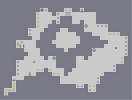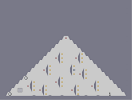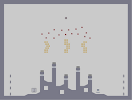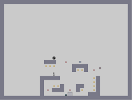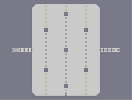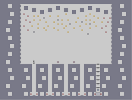04-4 05-0 05-1 05-2 05-3 05-4

Pages: (0)

### Wow

this Is quite cool, faved.

### Wow

this Is quite cool, faved.

### Well....

Fix the top. Refine the lines (check my latest map to see defined lines). Add a light (as said before me). Add more detail. It has potential, but for now, 2.5

### LIGHThouse

on top of the other ones, let there be light

### LIGHThouse

on top of the other ones, let there be light

### 

Try to make smoother lines for the lighthouse, and don't cut off the shading so quickly.